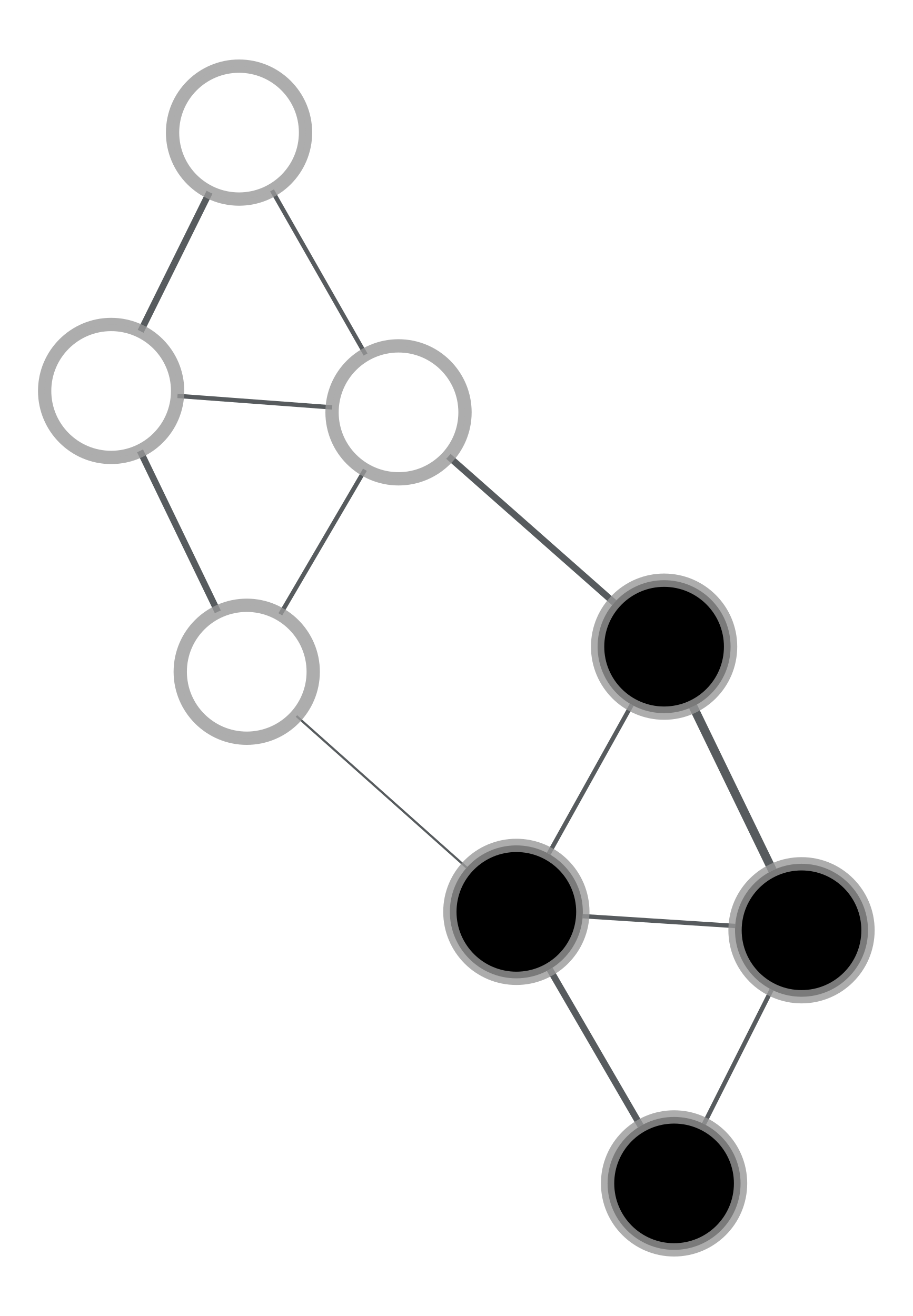# graph_tool.flow.min_cut#

graph_tool.flow.min_cut(g, weight)[source]#

Get the minimum cut of an undirected graph, given the weight of the edges.

Parameters:
gGraph

Graph to be used.

weightEdgePropertyMap

Edge property map with the edge weights.

Returns:
min_cutfloat

The value of the minimum cut.

partitionVertexPropertyMap

Boolean-valued vertex property map with the cut partition.

Notes

The algorithm is defined in [stoer_simple_1997].

The time complexity is $$O(VE + V^2 \log V)$$.

References

Stoer, Mechthild and Frank Wagner, “A simple min-cut algorithm”. Journal of the ACM 44 (4), 585-591, 1997. DOI: 10.1145/263867.263872 [sci-hub, @tor]

Examples

>>> g = gt.load_graph("mincut-example.xml.gz")
>>> weight = g.edge_properties["weight"]
>>> mc, part = gt.min_cut(g, weight)
>>> print(mc)
4.0
>>> pos = g.vertex_properties["pos"]
>>> gt.graph_draw(g, pos=pos, edge_pen_width=weight, vertex_fill_color=part,
...               output="example-min-cut.pdf")
<...>Vertices of the same color are on the same side of a minimum cut. The edge weights are represented by the edge width.#